# Banach space of analytic functions with infinite-dimensional domains

The primary interest here is in the interplay between function theory on infinite-dimensional domains, geometric properties of Banach spaces, and Banach and Fréchet algebras. Throughout,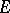will denote a complex Banach space with open unit ball.

## Definition and basic properties.

Let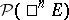denote the space of complex-valued-homogeneous polynomials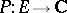, i.e. functions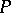to which is associated a continuous-linear functionsuch thatfor all. Each such polynomial is associated with a unique symmetric-linear form via the polarization formula. For an open subset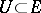, one says that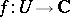is holomorphic, or analytic, ifhas a complex Fréchet derivative at each point of(cf. also Algebra of functions). Equivalently,is holomorphic if at each point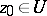there is a sequence of-homogeneous polynomials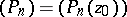such thatfor allin a neighbourhood of. If, then the algebra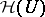of holomorphic functions from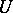to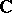always contains as a proper subset the subalgebraof holomorphic functions which are bounded on bounded subsetssuch that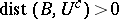. The latter space is a Fréchet algebra with metric determined by countably many such subsets, whereas there are a number of natural topologies on.

The natural analogues of the classical Banach algebras of analytic functions are the following: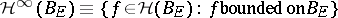;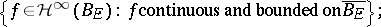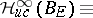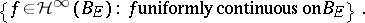All are Banach algebras with identity when endowed with the supremum norm (cf. also Banach algebra).

## Results and problems.

For any of the above algebrasof analytic functions, let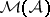denote the set of homomorphisms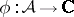. Since the Michael problem has an affirmative solution [a5], every homomorphism is automatically continuous. For each such, define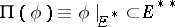(noting that, always,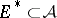). Basic topics of interest here are the relation between the "fibres"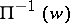,, and the relation between the geometry ofand of.

The spectrum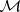displays very different behaviour in the infinite-dimensional setting, in comparison with the finite-dimensional situation. As an illustration, every element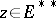corresponds to a homomorphism on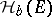. Indeed, for eachthere is a linear extension mapping from. Applying this mapping to the Taylor series of a holomorphic function yields a multiplicative linear extension operator, mapping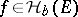to; similar results hold for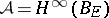and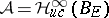. For example, eachyields an element of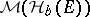via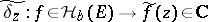. A complete description ofis unknown (1998) for general, although it is not difficult to see that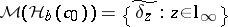. The question of whether the fourth dual ofalso provides points of the spectrum is connected with Arens regularity of[a7]. In any case,can be made into a semi-group with identity; the commutativity of this semi-group is related, once again, to Arens regularity of[a6].

It is natural to look for analytic structure in the spectrum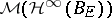. In fact, every fibreovercontains a copy of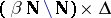. In many situations, e.g. whenis super-reflexive (cf., also Reflexive space), there is an analytic embedding of the unit ball of a non-separable Hilbert space into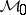. Further information has been obtained by J. Farmer [a8], who has studied analytic structure in fibres in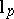-spaces. However, note that there is a peak set (cf. also Algebra of functions) for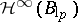which is contained in.

There has also been recent (1998) interest in the following areas:

reflexivity of;

algebras of weakly continuous holomorphic functions; and

Banach-algebra-valued holomorphic mappings.

Basic references on holomorphic functions in infinite dimensions are [a1], [a2], [a3]; a recent (1998) very helpful source, with an extensive bibliography, is [a4].